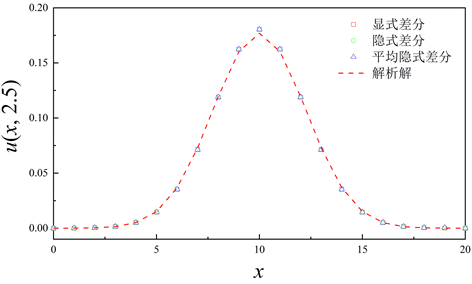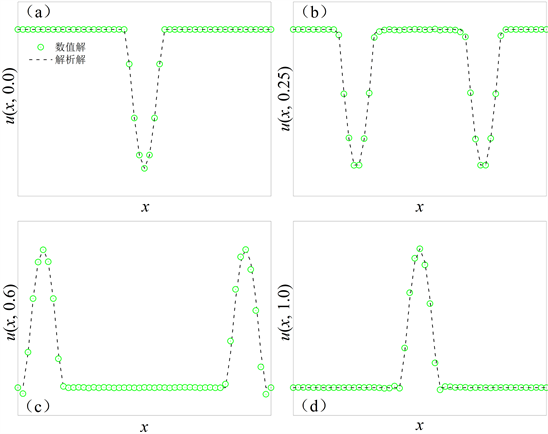﻿ 三类典型数理方程的数值解方法探析 Analysis on Numerical Solution Methods of Three Typical Mathematical Equations

Vol. 11  No. 01 ( 2022 ), Article ID: 48329 , 7 pages
10.12677/AAM.2022.111037Analysis on Numerical Solution Methods of Three Typical Mathematical Equations

Qiyuan Zhu

School of Mathematics, Physics and Big Data, Chongqing University of Science and Technology, Chongqing

Received: Dec. 24th, 2021; accepted: Jan. 14th, 2022; published: Jan. 26th, 2022ABSTRACT

Mathematical equations mainly refer to the partial equations derived from physical problems. As a professional basic course of engineering related majors, mastering the numerical solution method is necessary for students to pursue their careers. However, the main content of the course is the analytic method. Therefore, three kinds of typical equations are taken as examples, to discuss the ideas and methods of numerical solution by using the finite difference method. As verification and expansion of the main content of teaching, it is hoped to play an enlightening role in solving problems.

Keywords:Mathematical Equations, Numerical Methods, Finite Difference1. 引言

2. 一维扩散方程构成的定解问题

$\left\{\begin{array}{l}{u}_{t}-{a}^{2}{u}_{xx}=0\\ u\left(0,t\right)=0,\text{}u\left(l,t\right)=0\\ u\left(x,0\right)=\phi \left(x\right)\end{array}$ (1)

${u}_{t}\approx \frac{u\left(x,t+\Delta t\right)-u\left(x,t\right)}{\Delta t}$${u}_{xx}\approx \frac{u\left(x+\Delta x,t\right)-2u\left(x,t\right)+u\left(x-\Delta x,t\right)}{{\left(\Delta x\right)}^{2}}$

$u\left(x,t+\Delta t\right)\approx u\left(x,t\right)+\frac{\Delta t}{{\left(\Delta x\right)}^{2}}{a}^{2}\left[u\left(x+\Delta x,t\right)-2u\left(x,t\right)+u\left(x-\Delta x,t\right)\right]$

$r=\frac{\Delta t}{{\left(\Delta x\right)}^{2}}{a}^{2}$$x=i\Delta x$$t=j\Delta t$$\left(i,j=0,1,2,\cdots \right)$，上式可写为下标形式，即

${u}_{i,j+1}-{u}_{i,j}=r\left({u}_{i+1,j}-2{u}_{i,j}+{u}_{i-1,j}\right)$ (2)

${u}_{i,j+1}=\left(1-2r\right){u}_{i,j}+r\left({u}_{i+1,j}+{u}_{i-1,j}\right)$ (3)

${u}_{i,j+1}-{u}_{i,j}=r\left({u}_{i+1,j+1}-2{u}_{i,j+1}+{u}_{i-1,j+1}\right)$ (4)

$-r{u}_{i-1,j+1}+\left(1+2r\right){u}_{i,j+1}-r{u}_{i+1,j+1}={u}_{i,j}$ (5)

${\left(\begin{array}{cccccc}1+2r& -r& & & & \\ -r& 1+2r& -r& & & \\ & -r& 1+2r& & & \\ & & \ddots & \ddots & & \\ & & & -r& 1+2r& -r\\ & & & & -r& 1+2r\end{array}\right)}_{m-1,m-1}\left(\begin{array}{c}{u}_{1,j+1}\\ {u}_{2,j+1}\\ {u}_{3,j+1}\\ ⋮\\ {u}_{m-2,j+1}\\ {u}_{m-1,j+1}\end{array}\right)=\left(\begin{array}{c}{u}_{1,j}+r{u}_{0,j+1}\\ {u}_{2,j}\\ {u}_{3,j}\\ ⋮\\ {u}_{m-2,j}\\ {u}_{m-1,j}+r{u}_{m,j+1}\end{array}\right)$

$-\frac{r}{2}{u}_{i+1,j+1}+\left(1+r\right){u}_{i,j+1}-\frac{r}{2}{u}_{i-1,j+1}=\left(1-r\right){u}_{i,j}+\frac{r}{2}{u}_{i+1,j}+\frac{r}{2}{u}_{i-1,j}$ (6)

$A=\left(\begin{array}{cccccc}2\left(1+r\right)& -r& & & & \\ -r& 2\left(1+r\right)& -r& & & \\ & -r& 2\left(1+r\right)& & & \\ & & \ddots & \ddots & & \\ & & & -r& 2\left(1+r\right)& -r\\ & & & & -r& 2\left(1+r\right)\end{array}\right)$${u}_{j}=\left(\begin{array}{c}{u}_{1,j}\\ {u}_{2,j}\\ {u}_{3,j}\\ ⋮\\ {u}_{m-2,j}\\ {u}_{m-1,j}\end{array}\right)$

$B=\left(\begin{array}{cccccc}2\left(1-r\right)& r& & & & \\ r& 2\left(1-r\right)& r& & & \\ & r& 2\left(1-r\right)& & & \\ & & \ddots & \ddots & & \\ & & & r& 2\left(1-r\right)& r\\ & & & & r& 2\left(1-r\right)\end{array}\right)$${f}_{j}=\left(\begin{array}{c}r{u}_{0,j}\\ 0\\ 0\\ ⋮\\ 0\\ r{u}_{m,j}\end{array}\right)$

$A{u}_{j+1}=B{u}_{j}+{f}_{j}+{f}_{j+1}$ (7)

$l=20,\text{}{a}^{2}=1,\text{}t\in \left[0,2.5\right],\text{}\phi \left(x\right)=\left\{\begin{array}{cc}1& \left(9.5\le x\le 10.5\right)\\ 0& \left(x<10,\text{}x>11\right)\end{array}$

$u\left(x,t\right)=\underset{n=1}{\overset{\infty }{\sum }}\frac{2}{n\pi }\left(\mathrm{cos}\frac{n\pi }{2}-\mathrm{cos}\frac{11n\pi }{20}\right){\text{e}}^{-\frac{{n}^{2}{\pi }^{2}}{400}t}\mathrm{sin}\frac{n\pi x}{20}$Figure 1. Comparison of numerical solutions based on different difference formulas and analytical solutions at t = 2.5

3. 一维波动方程构成的定解问题

$\left\{\begin{array}{l}{u}_{tt}-{a}^{2}{u}_{xx}=0\\ u\left(0,t\right)=0,\text{}u\left(l,t\right)=0\\ u\left(x,0\right)=\phi \left(x\right),\text{}{u}_{t}\left(x,0\right)=\psi \left(x\right)\end{array}$ (8)

$\frac{u\left(x,t+\Delta t\right)-2u\left(x,t\right)+u\left(x,t-\Delta t\right)}{{\left(\Delta t\right)}^{2}}={a}^{2}\frac{u\left(x+\Delta x,t\right)-2u\left(x,t\right)+u\left(x-\Delta x,t\right)}{{\left(\Delta x\right)}^{2}}$

$r=\frac{{\left(\Delta t\right)}^{2}}{{\left(\Delta x\right)}^{2}}{a}^{2},\text{}x=i\Delta x,\text{}t=j\Delta t,\text{}\left(i,j=0,1,2,\cdots \right)$

${u}_{i,j+1}=r\left({u}_{i+1,j}+{u}_{i-1,j}\right)+2\left(1-r\right){u}_{i,j}-{u}_{i,j-1}$ (9)

$u\left(x,t\right)=\underset{n=1}{\overset{\infty }{\sum }}{C}_{n}\mathrm{cos}\frac{n\pi a}{l}t\mathrm{sin}\frac{n\pi }{l}x$

$\phi \left(x\right)=\left\{\begin{array}{ll}0.05\mathrm{sin}\left(7\pi x\right),\hfill & \frac{3}{7}$\psi \left(x\right)=0$Figure 2. Comparison of wave states obtained by numerical method and analytic formula at t = 0, 0.25, 0.6 and 1.0

4. 二维场位方程构成的定解问题

$\left\{\begin{array}{l}{u}_{xx}+{u}_{yy}=0,\text{}\left(0 (8)

${u}_{i,j}=\frac{1}{4}\left({u}_{i+1,j}+{u}_{i-1,j}+{u}_{i,j+1}+{u}_{i,j-1}\right)$ (9)

${u}_{i,j}=\left(1-\omega \right){u}_{i,j}+\frac{\omega }{4}\left({u}_{i+1,j}+{u}_{i-1,j}+{u}_{i,j+1}+{u}_{i,j-1}\right)$ (10)Figure 3. In the case of different relaxation factors, the function value at x = 50 (i.e. the position of the white dotted line in the illustration) was calculated with respect to y after 2000 iterations. The inset shows the distribution of the function u(x, y) after iterative convergence

5. 总结

Analysis on Numerical Solution Methods of Three Typical Mathematical Equations[J]. 应用数学进展, 2022, 11(01): 302-308. https://doi.org/10.12677/AAM.2022.111037

1. 1. 张静, 张彦, 唐琼, 等. “数学物理方法”课程改革的探索[J]. 大学教育, 2014(16): 163-165.

2. 2. 王正斌, 毛巍威, 杨志红. “数理方程”课程教学改革探索[J]. 宜春学院学报(自然科学), 2006, 28(6): 51-52.

3. 3. 刘鹏飞, 高博. 数学建模思想在数理方程教学中的渗透[J]. 吉林师范大学学报(自然科学版), 2007, 4(4): 132-133.

4. 4. 吕同富, 康兆敏, 方秀男. 数值计算方法[M]. 第2版. 北京: 清华大学出版社, 2013: 86.

5. 5. 彭芳麟. 计算物理基础[M]. 北京: 高等教育出版社, 2010: 346.

6. 6. 胡其华, 熊建明. 数理方程动边界问题研究的发展状况与主要研究方法[J]. 九江学院学报(自然科学版), 2005, 2(2): 87-89.